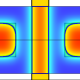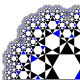# The Fundamental Dream of Algebra

According to modern pure mathematics, there is a basic fact about polynomials called “The Fundamental Theorem of Algebra (FTA)”. It asserts, in perhaps its simplest form, that if p(x) is a non-constant polynomial, then there is a complex number z which has the property that p(z)=0 . So every non-constant polynomial equation p(x)=0 has at least one solution. [NOT!]

When we combine this with Descartes’ Factor Theorem, we can supposedly deduce that a degree n polynomial can be factored into exactly n linear factors. [NOT!]

What a useful and crucially important theorem this is! It finds immediate application to integration, allowing us to integrate rational functions by factoring the denominators and using partial fractions to reduce all such to a few canonical forms. In linear algebra, the characteristic polynomial of a matrix has zeroes which are the eigenvalues. In differential equations or difference equations, these eigenvalues allow us to write down solutions. [NOT!]

Unfortunately, the theorem is a mirage. It is not really true. The current belief and dependence on the “Fundamental Theorem of Algebra” is a monumental act of collective wishful dreaming. In fact the result is not even correctly formulated to begin with. It is a false and idealized shadow of a much more complex, subtle and (ultimately) beautiful result.

Wait a minute Norman! Do we not have a completely explicit and clear formulation of the FTA? Are there not watertight proofs? Surely we have lots of concrete and explicit verifications of the theorem!?

Sadly the answers/responses are no in each case. The theorem is not correctly stated, because it requires a prior theory of complex numbers, which in turn require a prior theory of real numbers, and there is no such theory currently in existence, as you can get a pretty clear idea from by opening up a random collection of Calculus or Analysis texts. Or you could go through the fine-toothed comb dismantling of the subject in my MathFoundations YouTube series. And there are, contrary to popular belief, no watertight proofs. All current arguments rest on continuity assumptions that are not supported by proper theories, but ultimately only by appeals to physical intuition. And when we look at explicit examples, it quickly becomes obvious that the FTA is not at all true!

This issue confounded both Euler and Gauss–both struggled to give proofs, and both were defeated. Gauss returned over and over to the problem, each time trying to be more convincing, to be tighter, to overcome the subtle assumptions that can so easily creep into these arguments. He was not in fact successful.

Every few years some one comes up with a new “proof”, often unaware that the real difficulty is in framing the statement precisely in the first place! Unless you have a rock solid foundation for “real numbers”, all attempts to establish this result in its current form are ultimately doomed.

Most undergraduates learn from an early age to accept this theorem. Suspiciously, they are rarely presented with a proper proof. Sometime in a complex analysis course, if they get that far, they are exposed to a rather indirect argument involving Cauchy’s theory of integration. Are they convinced by this? I hope not: why should something so elementary have such a complicated proof? How do we know that some crucial circularity is not built into the whole game, if we only get around to “proving” a result in third year university that we have been happily assuming in all prior courses for years earlier?

And what about explicit examples? Is this not the way to sort out the wheat from the chaff?  Yes it is, and all we need to do is open our eyes clearly and look beyond our wishful dreaming to see things as they really are, not the way we would like them to be in our alternative Polyanna land of modern pure mathematics!

We write down a polynomial equation of some low degree. Let’s say to be explicit x^5-2x^2+x-7=0. Now I open my computer’s mathematical software package (in my case that is Scientific Workplace, which uses MuPad as its computational engine).

This program is no slouch– it has innumerably many times performed what are essentially miracles of computation for me. It can factor polynomials in several variables of high degree with hundreds, indeed thousands of terms. I have just asked it to factor the randomly typed number 523452545354624677876876875744666. It took about 3 seconds for it to determine that the prime factorization is

2 x 3 x 191 x 2033466852397 x 224623712 574400693 .

So let’s see what happens if I ask it to solve

p(x)=x^5-2x^2+x-7=0.

Actually that depends what kind of solving I ask for. If I ask for a numeric solution, it gives

{[x=-1. 166+1. 097i],[x=-1. 166-1. 097i],[x=0.3654-1. 254i],[x=0.3654+1. 254i],[x=1. 601]}.

Indeed we get 5 “solutions”, four consisting of two complex conjugate pairs, and one “real solution”. The reason we only get 4 digit accuracy is because of the current settings. Suppose I up the significant digits to 7. In that case, solve numeric returns

{[x=-1. 166064+1. 09674i],[x=-1. 166064-1. 09674i],[x=0.3654393-1. 253958i],[x=0.3654393+1. 253958i],[x=1. 601249]}.

Are these really solutions? No they are NOT. They are rational (in fact finite decimal) numbers which are approximate solutions, but they are not solutions. Let us be absolutely clear about this. Here for example is

p(-1. 166064+1. 09674i)=4. 164963586 717619097 5×10⁻⁶+9. 468394675 149678997 9×10⁻⁶ i

where I have upped the significant digits to 20, the maximum that is allowed. Do we get zero? Clearly we do NOT.

How about if I ask for solve exact solutions? In that case, my computer asserts that

Solution is: ρ₁ where ρ₁ is a root of Z-2Z²+Z⁵-7.

In other words, the computer is not able to find true exact solutions to this equation. The computer knows something that most modern pure mathematicians seem to be unaware of. And what is that? Come closer, and I will whisper the harsh truth to you..

This .. equation .. does .. not .. have .. exact .. solutions.

## 16 thoughts on “The Fundamental Dream of Algebra”

1.DJDizhna

Hi Norman,
Great article. Did you know that you can use LaTeX in WordPress? I’ve been using it for my blogs. I think you just type in$[insert code here]$, and it will automatically recognise this as LaTeX code.
Cheers,
G

1.DJDizhna

Whoops, that came out bad. Type in [dollar sign] latex [insert code here] [dollar sign].

2.Bob

So where do we go next?

3.gentzen

How about an interpretation of the theorem in the spirit of double negation in intuitionistic logic? (You won’t find an environment of 0 which doesn’t intersect the range of the polygon.) Then the proofs of FTA itself can stay mostly unchanged. The consequences are no longer as nice, and will get more complicated to state, but are overall still usable in principle.

1.njwildberger: tangential thoughts Post author

I agree that one could try to reformulate the theorem in this way, and that would be one way of trying to state it in terms of rational numbers and their associated complex numbers. However you still will have to wrestle with ambiguities: what for example do you mean by the range of the polynomial (I presume that is the word you meant)?

I don’t believe that the proofs of FTA will stay unchanged–something will have to be added. Currently it is not only the statement of the theorem which is problematic, but the proofs are too.

1.gentzen

You are right, the proofs of FTA must (most probably) be modified significantly. Luitzen Egbertus Jan Brouwer realized in 1908 that tertium non datur cannot be taken for granted, but only in 1924 he and B. de Loor were able to give an intuitionistically acceptable proof of FTA. But only in 1930, Heyting algebras were introduced by Arend Heyting to formalize intuitionistic logic, so maybe this doesn’t mean much. However, the work of Errett Bishop (or its posthumously updated revision) is probably more relevant to your goals than Brouwer’s intuitionism anyway, and it seems to be quite successful overall.

2.Dan

Luckily the normal version of FTA is already intuitionisticly valid. You can always construct via an algorithm a sequence of rational complex numbers such that when you put the nth value of the sequence into the polynomial, the abs of the output will be less than 1/n away from 0, e.g. the sequence is not apart from 0, which is the strongest form of equality.
This proof from Wikipedia can be made constructive by just using the intuitionistic weakening of the IVT.
https://en.m.wikipedia.org/wiki/Fundamental_theorem_of_algebra#Algebraic_proofs

1.njwildberger: tangential thoughts Post author

Thanks Jonathan, I find it curiously satisfying to see an approximate solution to such an equation with so many digits. Somehow I get a better idea of what is going on than just with 1.6012…

1.Jonathan Crabtree

1.6012^5-2*1.6012^2+1.6012-7 = -0.00134225334 not zero.

An estimate of either the real or complex solutions for x in x^5-2x^2+x-7=0 with hundreds of digits will still never get us to zero.

Yet we only need know pi to 32 places to calculate the size of the known universe within the accuracy of one proton. SOURCE: http://www.bbc.co.uk/programmes/p004y291 That’s the power of mathematics!

BTW Norman, I updated the applet to include insanely better approximations for the complex number solutions that will also never result in zero.

2.Jonathan Crabtree

I just realised we can type exponents. No more caret or hat ^ symbols!
x⁵ – 2x² + x – 7 = 0

4.joelsjogren

Wow! I didn’t know about these articles. One post on politics, this one about polynomial roots, one about your “boring seminars”, many current social aspects of math and my suspicion that the Banach–Tarski theorem is nonsense verified.

As a funny aspect of language, you can parse the last part of my sentence/list in two ways:
— my suspicion {that the Banach-Tarski theorem is nonsense} verified
— my suspicion that the Banach-Tarski theorem is {nonsense verified}
and they are independently true (though I haven’t read the whole thing yet).

5.Erick Anthony

Professor wildberger, how do you feel about the algebraic numbers? Not Geometrically, but just as objects? I think they are very beautiful

6.Anonymous

Why do you hate ZFC?

7.Bud Hammons

Like everyone else, I was taught the prevailing orthodoxy in mathematics. I was guilty of asking some uncomfortable questions in the process. After a lot of reflection, I think we should adopt the more realistic, modest approach: eschew infinite processes, non-constructive proofs, and embrace the truth that most problems in mathematics admit only approximate solutions, clearly delineate those conditions where exact solutions are feasible. I believe there are good and practical reasons to pursue this program: exhume or eliminate the possibility that the weaknesses in existing math foundations have led to problematic conclusions in physics and other “hard” sciences. Professor Wildberger has done us all a signal service in his quest to propagate this different approach to mathematics.

1.njwildberger: tangential thoughts Post author

Thanks Bud, it would be good to see more people considering moving to this more careful and ultimately sensible position.